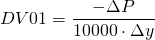# DV01

The DV01 or the dollar value of 1 basis point, also referred to as bpv or basis point value. This is a duration related metric in determining the interest rate sensitivity of a bond. The metric shows by how much the price in the bond changes by 1 basis point change in the interest rate. Its use is widespread among dealers and traders since they require to know the dollar amount of their portfolio on a daily basis. It’s less used in the context of portfolio management where the relative price changes are of more importance for portfolio hedging requirements.

# DV01 use and limitations

The DV01 of a portfolio can simply be calculated as the weighted average of all DV01 of every positions in which the weights represent the value of the position compared to the whole portfolio.

# How to calculate the DV01?

The DV01 value is calculated by taking the negative of the change in the bond price divided by 10.000 times the change in the underlying interest rate. The negative sign is the consequences of the negative relationship between bond prices and bond yields.## Summary

The DV01 metrics hels traders and investments managers to determine the absolute change of the portfolio / positions value if interest change by 1 bass point. Just likes duration it indicates the interest rate risk of a portfolio by measuring the linear relationship between bond prices and bond yields.